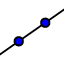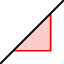# Graphing Linear Equations in Standard Form

Author:
garienj

## Graph the line -2x + 3y = -6

We can find the x and the y intercepts of the line by the following:
1. First, let .  This can be done by writing it out, but it is easier to just cover up the x-term with your finger as if it isn't even there.    Divide both sides by 3 to get .  Plot the point on the y-axis.  Click onand plot the point.
2. Next, let . Once again, it is easier to just cover up the y-term with your finger.   Divide both sides by -2 to get .  Plot the point on the x-axis.
3. Now, click on the iconin the menu to create a line the goes through both points.

## Find the slope of -2x + 3y = -6

Now that the line has been graphed, let's find the slope of the line.
1. Using the formula, , plug in the coordinates of the two points.
2. On the menu, click on the iconand then arrow down to the iconSlope.  Click on the line.  The slope will be displayed as a decimal rounded to two decimal places.